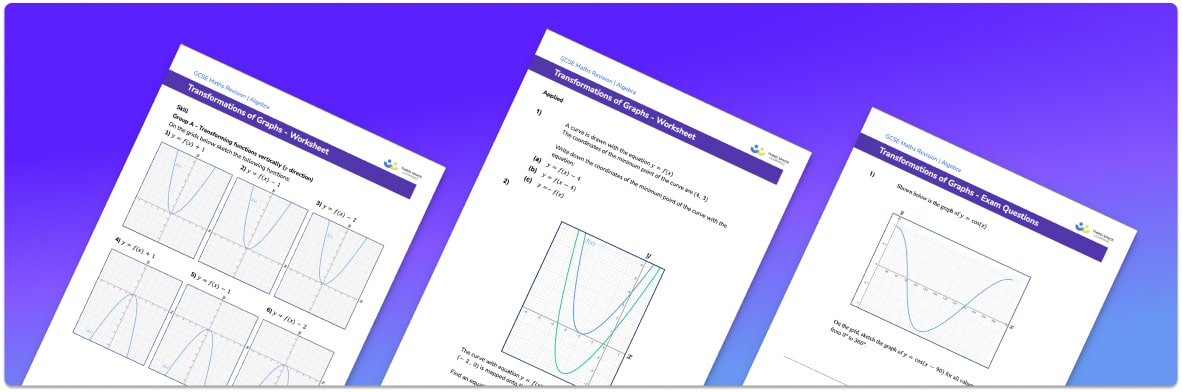# Transformations Of Graphs Worksheet• Section 1 of the transformations of graphs worksheet contains 20+ skills-based transformations of graphs questions, in 3 groups to support differentiation
• Section 2 contains 3 applied transformations of graphs questions with a mix of worded problems and deeper problem solving questions
• Section 3 contains 3 foundation and higher level GCSE exam style questions on transformations of graphs
• Answers and a mark scheme for all transformations of graphs questions are provided
• Questions follow variation theory with plenty of opportunities for students to work independently at their own level
• All questions created by fully qualified expert secondary maths teachers
• Suitable for GCSE maths revision for AQA, OCR and Edexcel exam boards

• This field is for validation purposes and should be left unchanged.

You can unsubscribe at any time (each email we send will contain an easy way to unsubscribe). To find out more about how we use your data, see our privacy policy.

### Transformations of graphs at a glance

Graph transformations are transformations that can be applied to graphs of functions. In GCSE maths, we study reflections and translations of graphs. Transforming a graph affects the equation of the graph in a specific way that we need to learn. If we start with the graph with equation y=f(x), then:

y=f(x)+a is a translation of a units in the y direction (vertical translation)

y=f(x+a) ia a translation of -a in the x direction (horizontal translation)

y=-f(x) is a reflection in the x axis

y=f(-x) is a reflection in the y axis

In GCSE maths, you may be asked to sketch a graph after identifying the transformation from the equation, or to write the equation after identifying the transformation. You may also be asked to write down the new coordinates of a certain point on the graph, for example the new coordinates of the minimum point or maximum point (also called the turning points) of the graph.

Graph transformations are studied further in A Level maths and enlargements of graphs (called stretches) are introduced.

Looking forward, students can then progress to additional interpreting graphs worksheets and other algebra worksheets, for example a sequences worksheet, simultaneous equations worksheet or straight line graphs worksheet.For more teaching and learning support on Algebra our GCSE maths lessons provide step by step support for all GCSE maths concepts.

## Do you have KS4 students who need more focused attention to succeed at GCSE?There will be students in your class who require individual attention to help them succeed in their maths GCSEs. In a class of 30, it’s not always easy to provide.

Help your students feel confident with exam-style questions and the strategies they’ll need to answer them correctly with our dedicated GCSE maths revision programme.

Lessons are selected to provide support where each student needs it most, and specially-trained GCSE maths tutors adapt the pitch and pace of each lesson. This ensures a personalised revision programme that raises grades and boosts confidence.

Find out more